# Force

Aristotle famously represented a force as anything that causes an object to undergo “unnatural motion”. Sir Isaac Newton was one of the first scientists to study gravity and force. Any kind of force is just a push or a pull. It can be described as a push or pull on an object.

## What is Force?Push or pull of an object is considered a force. Push and pull come from the objects interacting with one another. Terms like stretch and squeeze can also be used to denote force.
In Physics, force is defined as:

The push or pull on an object with mass causes it to change its velocity.

Force is an external agent capable of changing a body’s state of rest or motion. It has a magnitude and a direction. The direction towards which the force is applied is known as the direction of the force, and the application of force is the point where force is applied.

The Force can be measured using a spring balance. The SI unit of force is Newton(N).

 Common symbols: $$\begin{array}{l}\vec F , \, F \end{array}$$ SI unit: Newton In SI base units: kg·m/s2 Other units: dyne, poundal, pound-force, kip, kilo pond Derivations from other quantities: F = m a Dimension: LMT-2

### What are the Effects of Force?

In physics, motion is defined as the change in position with respect to time. In simpler words, motion refers to the movement of a body. Typically, motion can either be described as:

1. Change in speed
2. Change in direction

The Force has different effects, and here are some of them.

• Force can make a body that is at rest to move.
• It can stop a moving body or slow it down.
• It can accelerate the speed of a moving body.
• It can also change the direction of a moving body along with its shape and size.

## Force Videos

#### Force And Its Types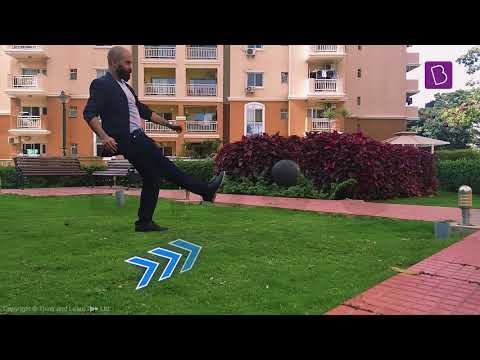#### Force – Push And Pull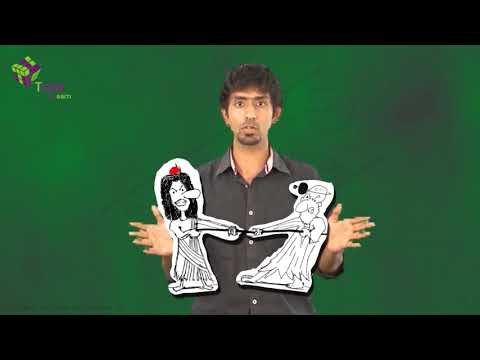#### Centripetal And Centrifugal Force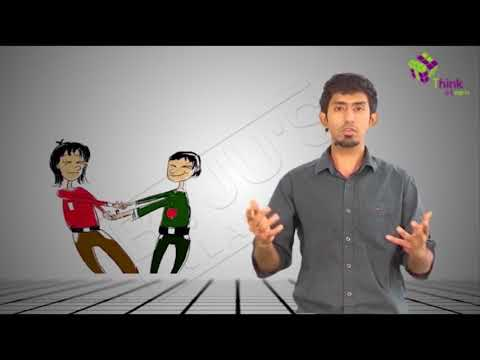#### Tension Force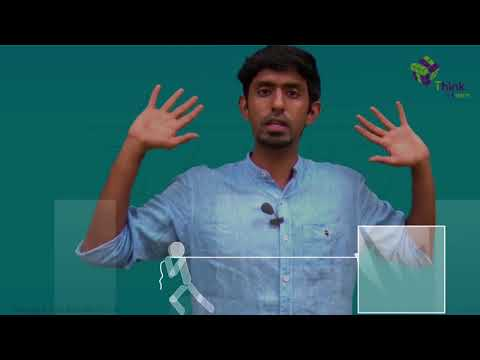You may also want to check out these topics given below!

## Formula for Force

The quantity of force is expressed by the vector product of mass (m) and acceleration (a). The equation or the formula for force can mathematically be expressed in the form of:

 F = ma

Where,

• m = mass
• a = acceleration

It is articulated in Newton (N) or Kgm/s2.

Acceleration a is given by

a = v/t

Where

• v = velocity
• t = time taken

So Force can be articulated as:

F = mv/t

Inertia formula is termed as p = mv which can also be articulated as Momentum.

Therefore, Force can be articulated as the rate of change of momentum.

F = p/t = dp/dt

Force formulas are beneficial in finding out the force, mass, acceleration, momentum, velocity in any given problem.

### Unit of Force

• In the centimeter gram second system of unit (CGS unit) force is expressed in dyne.
• In the standard international system of unit (SI unit) it is expressed in Newton (N).

## Types of Force

Force is a physical cause that can change an object’s state of motion or dimensions. There are two types of forces based on their applications:

1. Contact Force
2. Non-Contact Force

### Contact Force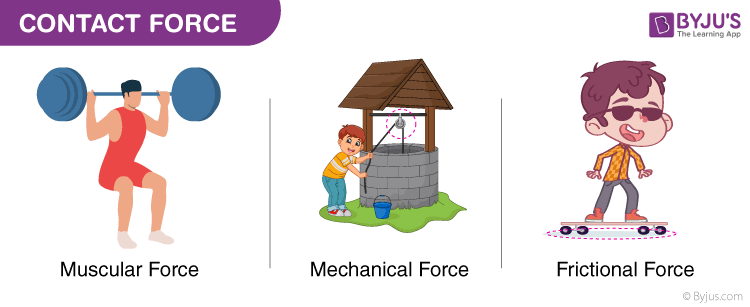Forces that act on a body either directly or through a medium are called contact forces.

Examples of contact forces are:

• Muscular Force
• Mechanical Force
• Frictional Force

We can use the muscular force of animals like bullocks, horses, and camels to get the activities done. The frictional force is another type of contact force, which acts between a pair of a surface in contact and tends to oppose the motion of one surface over the other.

### Non-Contact Force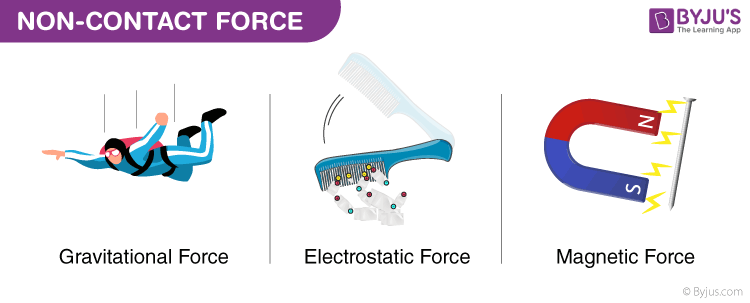Forces that act through spaces without making direct contact with the body are called non-contact forces.

Examples of non-contact forces are:

• Gravitational Force
• Electrostatic Force
• Magnetic Force

The force exerted by a magnet on other magnets is called magnetic force. Magnetic force and electrostatic force act on an object from a distance. That’s the reason they are non-contact forces. The strength of gravity is an attractive force that is exerted by the Earth on objects, which makes them fall to the land. The weight of a body is the force that is pulled by the earth towards the centre.

## Solved Examples

Q.1) How much net force is required to accelerate a 1000 kg car at 4.00 m/s2?

Solution:

Given,

• a = 4.00 m/s2
• m = 1000 kg

Therefore,

F = ma

= 1000 × 4

= 4000 N

Q.2) Aimee has a toy car mass of 2 kg. How much force should she apply to the car so that it should travel with the acceleration of 8 m/s2?

Solution:

Known,

• m (Mass of toy car) = 2 Kg,
• a (Acceleration) = 8m/s2,

F is Force to be applied by Aimmee = m × a

= 2 Kg × 8 m/s2 = 16 Kgm/s2  = 16 N.

Q.3) A hammer having a mass of 1 kg going with a speed of 6 m/s hits a wall and comes to rest in 0.1 sec. Compute the obstacle force that makes the hammer stop.

Solution:

Given,

• Mass of Hammer, m = 1 kg
• Initial Velocity, u = 6 m/s
• Final Velocity, v = 0 m/s
• Time Taken, t = 0.1 s

The acceleration is: a = (v – u)/t

Therefore, a =  -60

$$\begin{array}{l}m/s^{2}\end{array}$$

Thus, the retarding Force, F = ma = 1 × 60 = 60 N

## What is the Line of Action of a Force?

The line along which a force acts on an object is called the force’s line of action . The point where the force is acting on an object is called the point of application of the force. The force which opposes the relative motion between the surfaces of two objects in contact and acts along the surfaces is called the force of friction.

Galileo experimentally proved that objects that are in motion move with constant speed when there is no force acting on it. He could note that when a sphere rolls down an inclined plane, its speed increases because of the gravitational pull acting on it.

When all the forces acting on an object are balanced, the net force acting is zero. But, if all the forces acting on a body result in an unbalanced force, then the unbalanced force can accelerate the body, which means that a net force acting on a body can either change the magnitude of its velocity or change the direction of its velocity. For example, when many forces act on a body, and the body is found to be at rest, we can conclude that the net force acting on the body is zero.

## Frequently Asked Questions – FAQs

Q1

### Which is the weakest force in nature?

Gravity is the weakest force as its coupling constant is small in value.

Q2

### Which force is strongest?

The strongest force is the strong nuclear force which is 100 times stronger than the electromagnetic force.

Q3

### What are some types of forces?

Basically, there are two types of forces:

• Non-contact forces
• Contact forces
Q4

### What are some examples of force?

Some examples of force are:

• Gravitational force
• Electric force
• Magnetic force
• Nuclear force
• Frictional force
Q5

### Which force causes a charged balloon to attract another balloon?

Electrostatic force

Stay tuned with BYJU’S to learn more interesting science topics with engaging videos!

1. what are all the different types of force

• contact and non contact force

• cohesive and adhesive, surface tension, magnetic, gravitational force, tension force, frictional force, centripetal force, centrifugal force, electrostatics, upthrust, electrical force and nuclear force

2. nice examples thankyou.

3. Great Videos

4. Very useful I really appreciate it I could like to study with you people.

5. Nice exemples thank you so much I like your exemples

6. very helpful examples thank you

7. it is very usefulll i got 100/100 in all of my subjects after i started using byjus
thanks

8. Thank you

9. Thank you so much for the content! It really helped me a lot!

10. Thank you so much!This is very helpful.

11. It is very useful. I got 100/100 in all my subjects after I started using BYJU’S.
Thanks!

12. it really helped me in my physcis project thanks byjus

13. very nice information

14. It is a very excellent explanation of force.